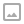## Projectile Reflection in Unity

·   ·  ☕ 2 min read

Sometimes things bounce. We’re going to put together a simple example of how to make projectiles do just that!

The formula for reflecting something off of a surface can be solved using only two inputs. The input vector and the normal direction of the surface that was hit. Once we have both of these values the reflection vector is equal to the input vector rotated 180 degrees around the normal vector. Luckily for us, Unity provides a built-in function for us so we don’t need to solve for this new reflected vector. Instead we can simply take advantage of Unity’s Vector3.Reflect function for this.

Now that we know how to reflect something we can build a recursive algorithm that allows us to dynamically tweak the maximum number of times that our vector can be reflected. Using raycasts we can determine if we hit anything and retrieve the information we need (where we hit and what direction it’s facing) then we can repeat and do it again. This gives us a basic simulation of projectile reflection that can be applied to most forms of projectiles you’d put in your game (we do it all at once here, but you can spread this out over multiple frames if needed).

Grab the code for this reflection simulation on GitHub here: https://gist.github.com/runewake2/ff564a39ba539a9bb1a71321c9f2723a

If you want to learn more about how projectiles can be reflected or specular light simulated this example covers the topic well and includes the formula’s you’ll need to calculate it yourself. http://ogldev.atspace.co.uk/www/tutorial19/tutorial19.html

Discord: https://discord.gg/hU5Kq2uWRITTEN BY
Sam Wronski
Maker of things, currently helping build cloud things @ Google. World of Zero is a personal project disconnected from my professional work. Lets make something awesome together!